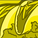# Water Cooler

This space is for everyone to ask questions related to the Community Platform. It's a space for us to get to know each other and have some fun! Come in and gather around the Water Cooler!

Announcements
QlikWorld 2023, a live, in-person thrill ride. Save \$300 before February 6: REGISTER NOW!
cancel
Showing results for
Did you mean:Contributor III

## Cumulative Sum by Year and Product in Script (QlikView)

Hi all,

I am trying to solve a problem in my load script of doing a cumulative sum but where I have multiple years and 3 products over which I want to sum.

My objective is to have a cumulative sum specific to a year and a product so I can compare the year by year cumulative figure.

I have been able to setup a cumulative sum in the script using this code:

``IF(Previous(CLIENT) = CLIENT,RangeSum(peek('RELN_iCNR_CUMULATIVE'), AMOUNT)) AS RELN_iCNR_CUMULATIVE​``

This works to calculate cumulative across the whole time period.

If I want to make this work within dimensions I tried this:

``IF(Previous(CLIENT) = CLIENTAND Previous(FACT_YEAR) = FACT_YEAR AND Previous(PRODUCT) = PRODUCT,RangeSum(peek('RELN_iCNR_CUMULATIVE_YR'), AMOUNT)) AS RELN_iCNR_CUMULATIVE_YR``

Which will work but gives a blank for the first month in a year.

My end point objective is to count where this cumulative sum > 1000 and compare this year by year. For instance if a client makes 500 revenue in Jan, count = 0, 500 in Feb, count = 1 (500+500) and continues as 1 for the rest of the year

Really appreciate any pointers. I may be over complicating this

Labels (1)
• ### QlikView

1 Solution

Accepted SolutionsContributor III
Author

In case anyone is here looking for the same, I managed to solve the issue from 2 logic steps.

1) The reason the cumulative sum was not working was initially to do with the order in which I sort the table to do the cumulative calculation. Once I looked at the raw data table and worked out the sequence it became clear. Quick tip here is to do a cumulative sum excluding the extra dimension (i.e. year in this case) and then sort the raw table by this value. That will show you how it is being sorted in practice.

2) Once 1) was solved I still had the issue of gaps for the first year in each section. Using the insight from here: https://community.qlik.com/t5/QlikView-App-Dev/Script-cumulative-sum-group-by/td-p/1810410 I was able to see a solution of adding an initial logic to say 'if current year is not the same as previous then return the value, else perform the cumulative sum' Resulting code below:

``````IF(FIN_FACT_YEAR <> Previous(FIN_FACT_YEAR),FIN_PROD_MONTHLY_iCNR,
IF(FIN_CLIENT_NBR = Previous(FIN_CLIENT_NBR) AND FIN_FACT_YEAR=Previous(FIN_FACT_YEAR),RangeSum(peek('RELN_iCNR_CUMULATIVE_LOGIC'), FIN_PROD_MONTHLY_iCNR))) AS RELN_iCNR_CUMULATIVE_LOGIC``````
3 RepliesMVP & Luminary

There is no else-branch within the if-loop. You may it trying in this way:

load *, -(RELN_iCNR_CUMULATIVE_YR >= 1000) as RevenueFlag;
IF(Previous(CLIENT) = CLIENT AND
Previous(FACT_YEAR) = FACT_YEAR AND
Previous(PRODUCT) = PRODUCT,
RangeSum(peek('RELN_iCNR_CUMULATIVE_YR'), AMOUNT),
AMOUNT) AS RELN_iCNR_CUMULATIVE_YR
resident Source
order by CLIENT, FACT_YEAR, PRODUCT;Contributor III
Author

In case anyone is here looking for the same, I managed to solve the issue from 2 logic steps.

1) The reason the cumulative sum was not working was initially to do with the order in which I sort the table to do the cumulative calculation. Once I looked at the raw data table and worked out the sequence it became clear. Quick tip here is to do a cumulative sum excluding the extra dimension (i.e. year in this case) and then sort the raw table by this value. That will show you how it is being sorted in practice.

2) Once 1) was solved I still had the issue of gaps for the first year in each section. Using the insight from here: https://community.qlik.com/t5/QlikView-App-Dev/Script-cumulative-sum-group-by/td-p/1810410 I was able to see a solution of adding an initial logic to say 'if current year is not the same as previous then return the value, else perform the cumulative sum' Resulting code below:

``````IF(FIN_FACT_YEAR <> Previous(FIN_FACT_YEAR),FIN_PROD_MONTHLY_iCNR,
IF(FIN_CLIENT_NBR = Previous(FIN_CLIENT_NBR) AND FIN_FACT_YEAR=Previous(FIN_FACT_YEAR),RangeSum(peek('RELN_iCNR_CUMULATIVE_LOGIC'), FIN_PROD_MONTHLY_iCNR))) AS RELN_iCNR_CUMULATIVE_LOGIC``````Contributor III
Author

Thanks Marcus - I like the flag option here I had not thought of that. I was going to do the calculation in the dashboard for this part but the flag may be cleaner and faster no doubt